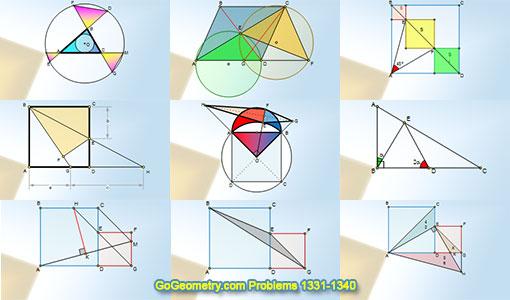# Geometry Problems 1331-1340. Level: High School, CollegeGeometry Problems 1331 - 1340Geometry Problem 1331. Two Squares Side by Side, Parallel, Perpendicular, 90 Degrees, Measurement. Geometry Problem 1332. Two Squares Side by Side, Triangle, Area. Geometry Problem 1333. Two Squares Side by Side, Three Triangles, Area, Measurement. Problem 1336. Right Triangle, Cevian, Double Angle, Perpendicular, 90 Degrees. Problem 1337. Parallelogram, Diagonals, Circle, Circumcircle, Tangent Line, Triangle, Congruence. Problem 1338. Four Squares, Diagonals, Angle, 45 Degrees, Areas. Problem 1339. Circle, Diameter, Tangent, Secant, Parallelogram. Problem 1340. Triangle, Incenter, Concentric Circles, Isosceles Triangles, Congruence.

Home | Sitemap | Geometry | Problems | Visual Index | 10 ProblemsEmail | Post a comment | by Antonio Gutierrez
Last updated: Feb 27, 2018